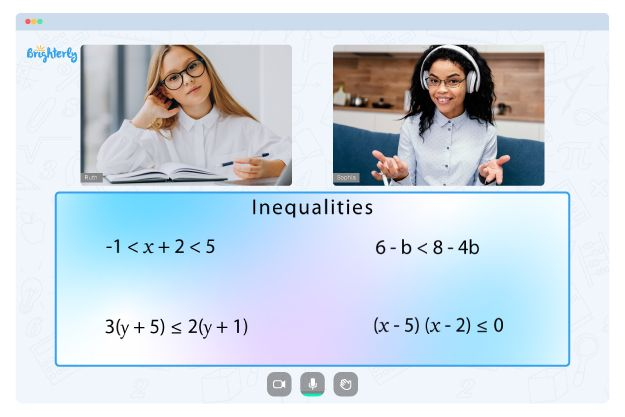# Graphing Linear Inequalities Worksheets

Linear inequalities are mathematical expressions that involve a linear function and an inequality symbol. The inequality symbol can be <, >, <=, or >=. The solution to a linear inequality is a set of values for the variable that makes the inequality true. The graphing linear inequalities worksheet helps students understand the concept easily.

## Importance of Graphing Linear Inequalities Worksheets

Graphing linear inequalities worksheet answers is an important tool for understanding and solving mathematical problems. Below are some of its benefits:

• Graphing linear inequalities is an important tool for visualizing and understanding the solution set of an inequality. It allows you to see the relationship between the inequality and the variables it involves.
• Graphing linear inequalities can also be useful in real-world situations. For example, the concept can be used in economics to graph supply and demand.
• Graphing linear inequalities also play a vital role in solving systems of linear inequalities which involve multiple inequalities and variables. Graphing a system of linear inequalities allows you to see the overlap or intersection of the solution sets and to identify the specific values of the variables that satisfy all of the inequalities in the system.

Math for Kids

Is Your Child Struggling With Math?
1:1 Online Math Tutoring## Benefits of Graphing Linear Inequalities Worksheet

Our graphing linear inequalities worksheet will help students learn a variety of important concepts and skills related to graphing linear inequalities. They include:

• How to write an inequality in slope-intercept form: With this worksheet, you’ll learn how to rewrite an inequality in the form y = mx + b, where m is the slope and b is the y-intercept.
• How to find the slope and y-intercept: Students will learn how to find the slope and y-intercept of an inequality, which are important steps for plotting the inequality on a coordinate plane.
• How to graph a linear inequality: Students will also learn how to plot the inequality on a coordinate plane and how to use the inequality symbol to determine which side of the line to shade.
• How to interpret the solution set of an inequality: Using our graph linear inequalities worksheet, students will learn how to read and interpret the solution set of an inequality and understand the relationship between the inequality and the variables it involves.

Our graphing linear inequalities worksheet with answers comes with detailed instructions and steps on solving linear inequalities problems. You’ll also have access to graphing linear inequalities shading the solution area worksheet answers as well as our sketch the graph of each linear inequality worksheet answers.### Graphing Linear Inequalities Worksheet PDF

Graph Linear Inequalities Worksheet### Graphing Linear Inequalities Worksheet PDF### Graphing Linear Inequalities Worksheet PDF

Graphing Linear Inequalities Worksheet With Answers### Graphing Linear Inequalities Worksheet PDF

Graphing Linear Inequalities Worksheet

Need help with Inequalities?• Does your child struggle to grasp of inequalities?
• Try lessons with an online tutor.

Is your child having difficulties with understanding inequalities? An online tutor could provide the necessary support.